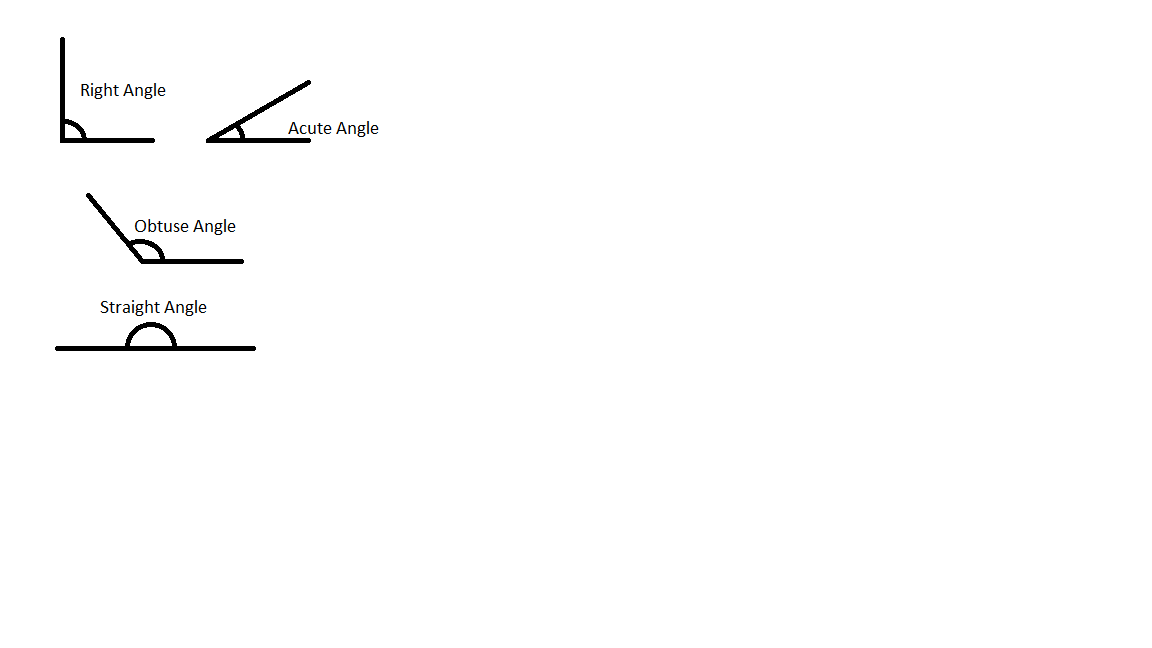## Basic College Mathematics (9th Edition)

Acute Angle: Angles that are less than 90 $^{\circ}$ Obtuse Angle: Angles that are greater than 90$^{\circ}$ Straight Angle: Angles equal to 180$^{\circ}$ Right Angle: Angles equal to exactly 90$^{\circ}$Acute Angle: Angles that are less than 90 $^{\circ}$ Obtuse Angle: Angles that are greater than 90$^{\circ}$ Straight Angle: Angles equal to 180$^{\circ}$ Right Angle: Angles equal to exactly 90$^{\circ}$